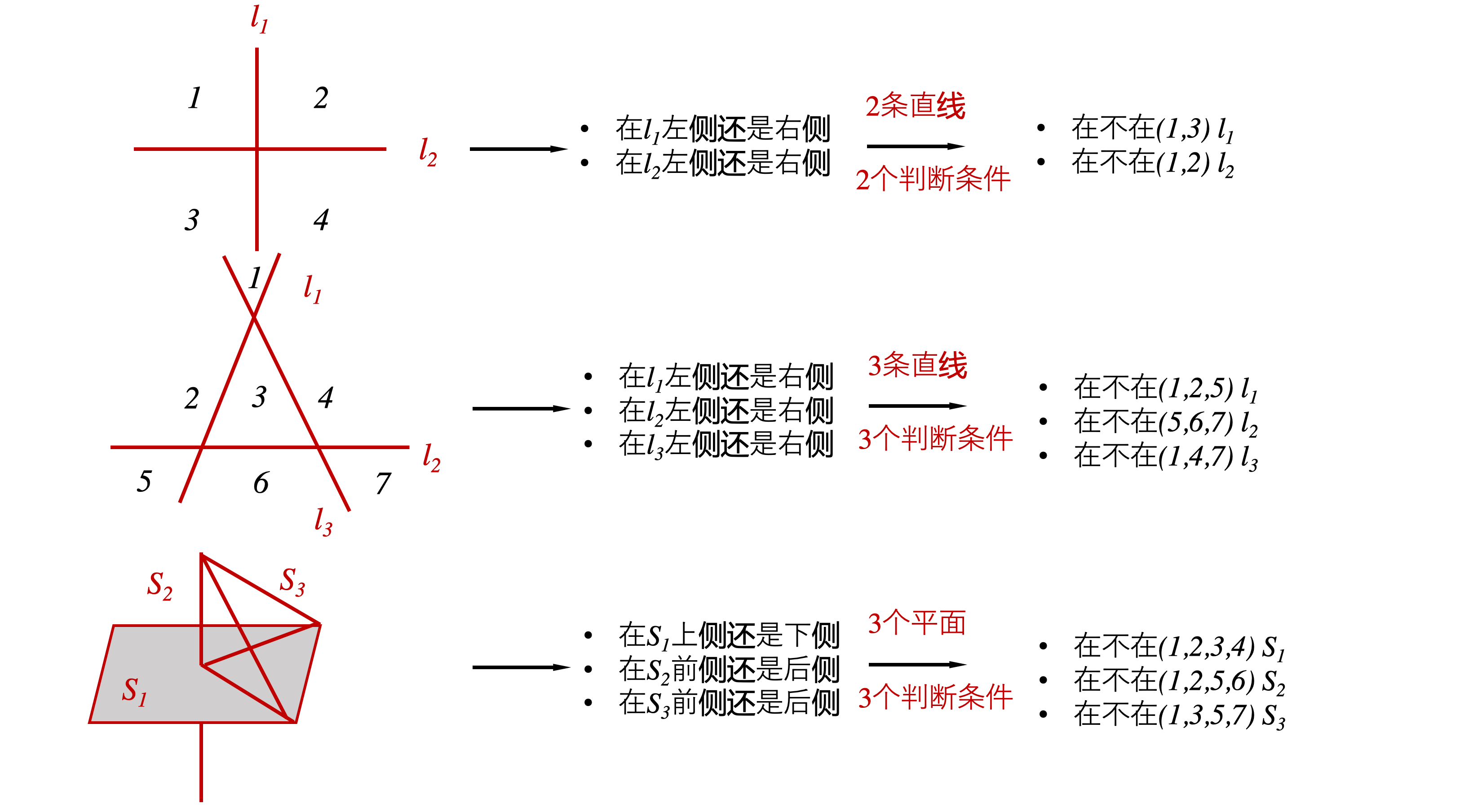# DeeCamp 2019 Exam A 纪实

DeeCamp Exam A 2019-04-27

• blank: 5
• QA: 1
• Time: 90min

update 一下 评论 已经有 dalao 给出第八题 思路 感谢 @熊怡 dalao

d 维空间中 n 刀切蛋糕最多能切多少块？求 f(d,n)## 1

A 0.9 0.9
B 0.9 0.7
C 0.7 0.9
D 0.7 0.7

1TPFN
0FPTN

p = T P T P + F P p=\frac{TP}{TP+FP}
r = T P T P + F N r=\frac{TP}{TP+FN}

1. 若 TP 数量不变，则 P 变大，r 不变或者减少
2. 若 TP 数量减少,则 p = T P T P + F P = 1 − 1 T P / F P + 1 p=\frac{TP}{TP+FP}=1-\frac{1}{TP/FP+1}
• 可以看出 p 与 T P F P \frac{TP}{FP} 成正相关
• T P F P \frac{TP}{FP} 增大时，p 增大
• 反之，p 减小
• 此情况下 recall 均会减小

## 2

A f ( z ) = max ⁡ ( 0 , 1 − z ) f(z)=\max (0,1-z)
B f ( z ) = z 3 f(z)=z^{3}
C f ( z ) = exp ⁡ ( − z ) f(z)=\exp (-z)
D f ( z ) = log ⁡ ( 1 + exp ⁡ ( − z ) ) f(z)=\log (1+\exp (-z))

## 3

GoogleNet 中 1 × 1 卷积的作用
A 降维
B 减少参数
C 跨通道通信
D 以上三项都对

## 4

Y = { 1 ,  if  w ∗ X + b &gt; 0 0 ,  otherwise  Y=\left\{\begin{array}{ll}{1,} &amp; {\text { if } w * X+b&gt;0} \\ {0,} &amp; {\text { otherwise }}\end{array}\right.

A 2
B 3
C 4
D 大于 4

## 5

A 1334323 / 1679616
B 1334324 / 1679616
C 1334325 / 1679616
D 1334326 / 1679616

import random
def foo(n):
x = 6
y = 1000000
count = 0
for i in range(n):
cur = y
print(i)
while cur > 0:
i = random.randint(0, x - 1)
if i > cur % x:
break
elif i < cur % x:
count += 1
break
cur = cur // x
return count /n


• 当采样值 > cur % 6，停止采样
• 当采样值 < cur % 6, count += 1 停止采样
• 当采样值 == cur % 6, cur = cur // 6，继续采样

• 1000000 % 6 = 4
• 166666 % 6 = 4
• 27777 % 6 = 3
• 4629 % 6 = 3
。。。

def mean():
begin = 1000000
cur = begin
count = 0
num = 0.0
while cur > 0:
temp = cur % 6
num += ((temp) / 6) * pow((1/6), count)
count += 1
cur = cur // 6
return num


## 7

X = [ 0.6 , 1.8 , 3.2 ] X=[0.6,1.8,3.2] ,输出多少

（感觉回到了高中 hhh

• W [ 1 , 2 , 3 ] + b = 1 W[1,2,3]+ b=1
• W [ − 1 , 1 , 4 ] + b = 2 W[-1,1,4]+ b=2
• W [ 0.6 , 1.8 , 3.2 ] + b = ? W[0.6, 1.8, 3.2] + b=?

m [ 1 , 2 , 3 ] + n [ − 1 , 1 , 4 ] = k [ 0.6 , 1.8 , 3.2 ] m[1,2,3]+n[-1, 1, 4] = k[0.6, 1.8, 3.2]

=> 4 M + N 5 = W [ 0.6 , 1.8 , 3.2 ] + b = 1.2 \frac{4 M+ N}{5}=W[0.6, 1.8, 3.2] + b=1.2

## 8

1. 你有 888 位微信好友
2. 你有多部手机，每部手机对应一个微信账号，你可以任意分配组合每部手机里面的微信好友（不限数量，可重复）
3. 一篇推荐的文章最多只被一位好友阅读
问； 现在你被推荐了一篇文章，在不删除好友、不进行变更好友组合的前提下，至少需要几部手机才能才能太通过便览各个手机的文章显示情况就能知道谁在看这篇文章

d 维空间中 n 刀切蛋糕最多能切多少块？求 f(d,n)0 一定是低位，然后依次推

## 10

MNIST 6w × 28 × 28 的手写字母数据集

DataSet: 链接:https://pan.baidu.com/s/1RK1Yz1hVSnFaogRsZHZ0Eg 密码:o6ui

from  numba import jit
x_train = data['x_train']
y_train = data['y_train']
crops = data['crops']
return x_train, y_train, crops

@jit
picture_list = []
for ii in x_train:
picture_list.append(ii[0:14, 0:14].copy())
picture_list.append(ii[14:28, 0:14].copy())
picture_list.append(ii[0:14, 14:28].copy())
picture_list.append(ii[14:28, 14:28].copy())
return picture_list

# @jit
def search(picture_list, corps):
result = []
for ii, jj in enumerate(picture_list):
for kk, mm in enumerate(crops):
if (jj == mm).all():
result.append((ii, kk, y_train[ii // 4]))
# print(ii, kk, y_train[ii // 4])
return result

def judge():
start = time.time()
result = search(picture_list, corps)
result_id = [ii for ii in result]
result_num_pre = [ii for ii in result_id if ii +
1 in result_id and (ii + 2 in result_id) and (ii + 3 in result_id)]
result_num = [y_train[ii//4] for ii in result_num_pre]
print(result_num)
print('{:.2f}s'.format(time.time() - start))


06-081792
06-04109108-20546
09-182798
09-01329
12-011362
01-28392
04-27
04-08253